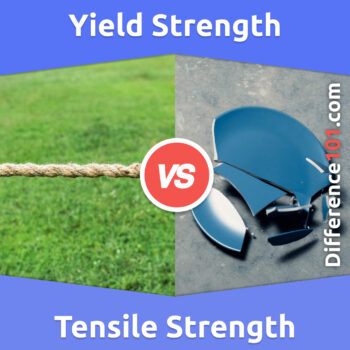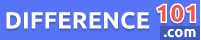# Yield Strength vs. Tensile Strength: What’s The Difference Between Yield Strength And Tensile Strength?

What’s the difference between yield strength and tensile strength? They are both important measures of strength, but they are not the same. This article will explain the difference between the two and why they are both important.Yield Strength vs. Tensile Strength are common words you have often heard in physics. Both of these terms are used to measure the strength of a material, but these are also different from each other. The main difference between yield strength and tensile strength is that under yield strength, a material changes its form completely, while tensile strength is the stress which a material can handle before it breaks.

Let’s take a closer look at Tensile Strength vs. Yield Strength

## What Is Yield Strength?If we look at the yield strength meaning: it can be defined as the minimum amount of force that is needed to deform something permanently. It can also be defined as a “measure of the strength of an object”. The deformation caused by yield strength is irreversible, which means the material can not be back into its previous shape. Which is also a basic difference between yield strength and tensile strength. Moreover, the stress which is applied in yield stress is minimum. Another term related to yield stress is used in Physics, known as “Elastic Limit”.Before reaching the yield strength, damage which is caused to a material can be reversed, which is called elastic deformation. But after the elastic limit is reached, the damage is beyond repair, and it is called plastic deformation.

👉  i5 vs. i7: What’s The Difference Between i5 And i7?

## What Is Tensile Strength?If we look at tensile strength meaning: it is the maximum amount of stress which bears by an object before it breaks. Tensile strength has a specific application in the field of engineering and physics. Tensile strength is an intensive property, as it does not depend upon the size of the object which is used. The tensile strength limit comes after the elastic limit. The difference between tensile and yield strength depends upon a few factors. As yield strength is the minimum force, it causes the deformation of that object permanently. While tensile is the maximum force that an object can bear before it starts breaking. So, in this case, the force which is applied externally is greater than the forces of attraction binding that object together.

👉  Mass vs. Weight: What Is The Difference Between Mass And Weight?

## Yield Strength vs. Tensile Strength Similarities

• Whether it is yield strength or tensile strength, both terms are used to measure the strength of a material.
• Both of these terms are used in physics and engineering and have great significance.
👉  Homo Erectus vs. Homo Sapien: What’s The Difference Between Homo Erectus And Homo Sapien?

## Yield Strength vs. Tensile Strength Examples

• Rope
• Wire
• Steel

### Tensile Strength Examples

• Utensils
• Stripping Piece of Paper
• Pulling water from the well

## Yield Strength vs. Tensile Strength Pros and Cons

### Yield Strength Pros and Cons#### Pros of Yield Strength

• The knowledge of yield strength is very important while designing the components since it represents the upper limit of load that can be applied.
• It is also very important in controlling material production techniques, such as pressing, rolling and forging.

#### Cons of Yield Strength

• Yield strength causes a permanent deformation, which means that the object can not come into its original shape ever.
• The hydrostatic pressure and temperature can easily affect yield strength and can even result in loss of force.

### Tensile Strength Pros and Cons#### Pros of Tensile Strength

• Tensile strength is very important for materials in order to determine their mechanical performance.
• Tensile strength also has great significance in engineering, as it allows engineers to decide how and when to use the material.

#### Cons of Tensile Strength

• Tensile strength is the maximum amount of force, which means it needs a lot of force to break an object.
• The preparation of the sampling in tensile testing can be very time-consuming and expensive.

## Comparison Chart## Conclusion

Yield strength and tensile strength are both important terms used in physics and engineering. These both refer to the strength of a material. But if we talk about yield strength vs. tensile strength differences, both work in different ways. As yield strength is the minimum amount of force to deform a material permanently. On the other hand, tensile strength is the maximum amount of force which an object can bear before it starts breaking. In yield strength, The intermolecular forces of the object are higher than the outer forces applied to it. While in tensile strength, The intermolecular or internal forces of the objects are weaker than the outer forces. And if we compare yield strength versus tensile strength numerical value. The yield strength has a lesser numerical value than tensile strength.Alex Stantor

Alex Stantor is a Sorbonne University (Paris, France) graduate in Philosophy and Data Analysis. Currently, he is an Author and Researcher at Difference 101, he writes articles/blog posts on topics such as "thinking differently" and "the importance of difference". Alex is a passionate advocate of diversity in the workplace and in companies, and diversity and inclusion in corporate communications. He currently lives in Brooklyn.

We will be happy to hear your thoughts